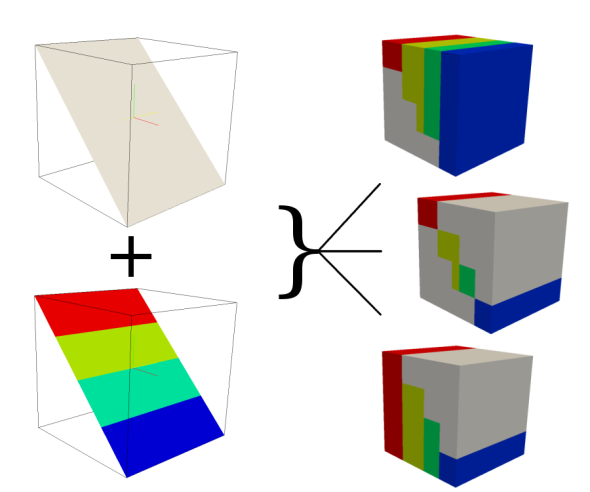## NAME

r.to.rast3elev - Creates a 3D volume map based on 2D elevation and value raster maps.

## KEYWORDS

raster, conversion, raster3d, voxel

## SYNOPSIS

r.to.rast3elev
r.to.rast3elev --help
r.to.rast3elev [-ulm] input=name[,name,...] elevation=name[,name,...] output=name [upper=float] [lower=float] [tilesize=integer] [--overwrite] [--help] [--verbose] [--quiet] [--ui]

### Flags:

-u
Use the input map values to fill the upper cells
-l
Use the input map values to fill the lower cells
-m
Use 3D raster mask (if exists) with input map
--overwrite
Allow output files to overwrite existing files
--help
Print usage summary
--verbose
Verbose module output
--quiet
Quiet module output
--ui
Force launching GUI dialog

### Parameters:

input=name[,name,...] [required]
Name of input raster map(s)
elevation=name[,name,...] [required]
Name of input elevation raster map(s)
output=name [required]
Name for output 3D raster map
upper=float
The value to fill the upper cells, default is null
lower=float
The value to fill the lower cells, default is null
tilesize=integer
The maximum tile size in kilo bytes. Default is 32KB.
Default: 32

## DESCRIPTION

Creates a 3D volume map based on 2D elevation and value raster maps. If the 2d and 3d region settings are different, the 2d resolution will be adjust to the 3d resolution.How r.to.rast3elev works

## NOTES

The hight of the 2D elevation maps will be used to verify the position within the 3D region. If the cell value of the elevation raster maps is located within the 3D region, the cell value of the appropriate 2D input raster maps will be written to the associated 3D cell. There are flags and options to fill the upper and lower 3D cells with a specific value, or the input raster maps values.

## Example

Simple Spearfish sample data set example
```g.region raster=elevation.10m -p
g.region res=200 res3=200 t=2000 b=0 tbres=20 -p

# Write the values of raster map soils based on the elevation of elevation.10m
# to the 3D map volev

r.to.rast3elev in=soils elev=elevation.10m out=volev

# Write the values of map soils based on the elevation of elevation.10m
# to the 3D map volev_l and fill the lower cells with the soils map values

r.to.rast3elev in=soils elev=elevation.10m out=volev_l -l

# Write the values of map soils based on the elevation of elevation.10m
# to the 3D map volev_u and fill the upper cells with the soils map values

r.to.rast3elev in=soils elev=elevation.10m out=volev_u -u

# Example with multiple elevation maps.
## first we need three support maps

r.mapcalc expression="one = 1"
r.mapcalc expression="two = 2"
r.mapcalc expression="three = 3"

## Now we generate the new evelation maps

r.mapcalc expression="elev_mid = elevation.10m - 500"
r.mapcalc expression="elev_bottom = elevation.10m - 1000"

## Now fill the lower cells below the elevation maps with the values one, two and three

r.to.rast3elev -l input=one,two,three elevation=elevation.10m,elev_mid,elev_bottom output=threelayer

## Export the map for visualization with paraview (http://www.paraview.org)
## By default the null value is -9999.99, we adjust it to 0.0 for
## better visualization
r3.out.vtk null=0.0 input=threelayer output=/tmp/threelayer.vtk

# Start paraview

paraview --data=/tmp/threelayer.vtk

# Note: First you need to choose the surface representation style and
# then color by "threelayer" in paraview.
```